Warning

This documents an unmaintained version of NetworkX. Please upgrade to a maintained version and see the current NetworkX documentation.

# expected_degree_graph¶

expected_degree_graph(w, seed=None, selfloops=True)[source]

Return a random graph with given expected degrees.

Given a sequence of expected degrees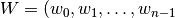) of length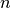this algorithm assigns an edge between node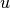and node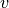with probability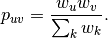Parameters: w (list) – The list of expected degrees. selfloops (bool (default=True)) – Set to False to remove the possibility of self-loop edges. seed (hashable object, optional) – The seed for the random number generator. Graph

Examples

>>> z=[10 for i in range(100)]
>>> G=nx.expected_degree_graph(z)


Notes

The nodes have integer labels corresponding to index of expected degrees input sequence.

The complexity of this algorithm is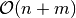whereis the number of nodes and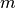is the expected number of edges.

The model in  includes the possibility of self-loop edges. Set selfloops=False to produce a graph without self loops.

For finite graphs this model doesn’t produce exactly the given expected degree sequence. Instead the expected degrees are as follows.

For the case without self loops (selfloops=False),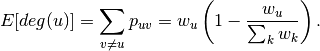NetworkX uses the standard convention that a self-loop edge counts 2 in the degree of a node, so with self loops (selfloops=True),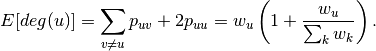References

  Fan Chung and L. Lu, Connected components in random graphs with given expected degree sequences, Ann. Combinatorics, 6, pp. 125-145, 2002.
  Joel Miller and Aric Hagberg, Efficient generation of networks with given expected degrees, in Algorithms and Models for the Web-Graph (WAW 2011), Alan Frieze, Paul Horn, and Paweł Prałat (Eds), LNCS 6732, pp. 115-126, 2011.